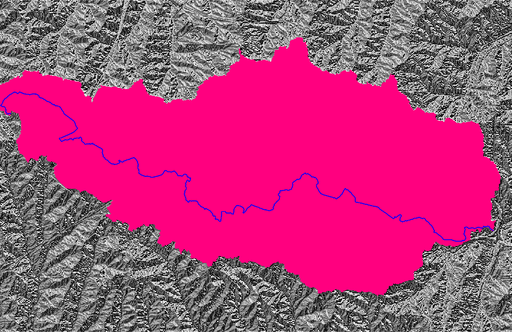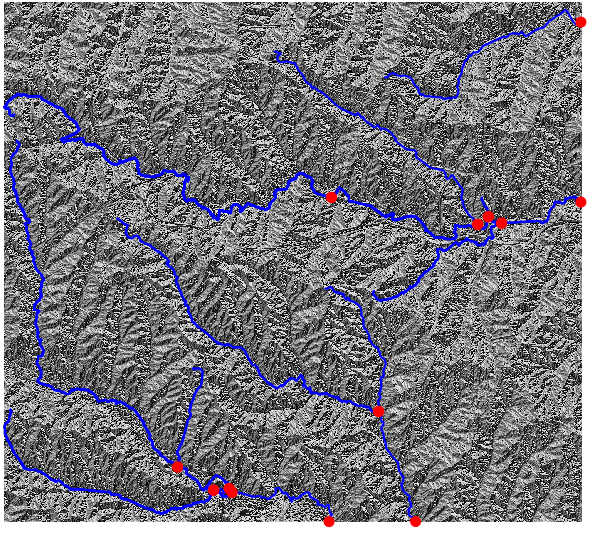## NAME

r.lfp - Calculates the longest flow paths for given outlet points using the r.stream.distance addon.

## KEYWORDS

hydrology, watershed

## SYNOPSIS

r.lfp
r.lfp --help
r.lfp input=name output=name [id_column=name] [id=integer[,integer,...]] [coordinates=east,north[,east,north,...]] [outlet=name] [layer=string] [outlet_id_column=name] [--overwrite] [--help] [--verbose] [--quiet] [--ui]

### Flags:

--overwrite
Allow output files to overwrite existing files
--help
Print usage summary
--verbose
Verbose module output
--quiet
Quiet module output
--ui
Force launching GUI dialog

### Parameters:

input=name [required]
Name of input drainage direction raster map
output=name [required]
Name for output longest flow path vector map
id_column=name
Name for output longest flow path ID column
id=integer[,integer,...]
ID for longest flow path
coordinates=east,north[,east,north,...]
Coordinates of outlet point
outlet=name
Name of outlet vector map
Or data source for direct OGR access
layer=string
Layer number or name
Vector features can have category values in different layers. This number determines which layer to use. When used with direct OGR access this is the layer name.
Default: 1
outlet_id_column=name
Name of longest flow path ID column in outlet map

## DESCRIPTION

r.lfp calculates the longest flow paths for given outlet points and creates a new vector map with those lines. This module requires the r.stream.distance addon.

## NOTES

r.lfp creates a longest flow path vector map using a drainage direction raster map and the coordinates of outlet points. The module internally runs r.stream.distance to calculate a flow length downstream raster map and r.path to trace the longest flow path from headwater cells. An input drainage map can be created using r.watershed or r.stream.extract.

r.lfp can read outlet coordinates from the coordinates and/or outlet parameters. For multiple outlets, the user can assign a unique ID to each longest flow path per outlet point using id, id_column, and outlet_id_column parameters. The id parameter specifies unique IDs for all outlets given in the coordinates parameter in the same order. The outlet_id_column specifies a column in the outlet map that contains unique IDs to be copied over to the id_column column in the output map.

## EXAMPLES

These examples use the North Carolina sample dataset.

Create the longest flow path for one outlet:

```# set computational region
g.region raster=elevation -p

# calculate drainage directions
r.watershed elevation=elevation drainage=drain_directions

# calculate outlet point related watershed
r.water.outlet input=drain_directions output=basin coordinates=642455,222614

# calculate longest flow path
r.lfp input=drain_directions output=lfp coordinates=642455,222614
```Note that there can be more than one longest flow path when multiple paths have the same flow length. In fact, the above example produces two lines with the same length.There are different ways to calculate multiple longest flow paths in one run:

```# calculate longest flow paths at two outlets
r.lfp input=drain_directions output=lfp coordinates=642455,222614,642306,222734

# calculate longest flow paths at two outlets and assign IDs
r.lfp input=drain_directions output=lfp coordinates=642455,222614,642306,222734 \
id=1,2 id_column=lfp_id

# calculate longest flow paths at all points in the outlets map
r.lfp input=drain_directions output=lfp outlet=outlets

# calculate longest flow paths at all points in the outlets map and assign IDs using a column in this map
r.lfp input=drain_directions output=lfp outlet=outlets \
id_column=lfp_id outlet_id_column=outlet_id

# calculate longest flow paths at given coordinates and all points in the outlets map and assign IDs
r.lfp input=drain_directions output=lfp coordinates=642455,222614,642306,222734 outlet=outlets \
id=1,2 id_column=lfp_id outlet_id_column=outlet_id
```

Calculate the longest flow paths for sub-watersheds:

```# get nsres
eval `r.info -g map=elevation`

# delineate streams using a threshold
r.stream.extract elevation=elevation threshold=50000 stream_vector=streams

# populate stream lengths
v.to.db map=streams option=length columns=length

# create points along the streams starting from downstream
v.to.points -r input=streams output=stream_points dmax=\$nsres

# find outlets (downstream-most less nsres points)
cats=`db.select -c sql="select stream_points_2.cat from stream_points_2 \
inner join stream_points_1 on stream_points_1.cat = stream_points_2.lcat \
where length-along > 0.5*\$nsres and length-along < 1.5*\$nsres"`
cats=`echo \$cats | tr " " ,`
v.extract input=stream_points layer=2 cats=\$cats output=stream_outlets

# create the longest flow paths for all outlets
r.lfp input=drain_directions output=lfp id_column=id outlet=stream_outlets layer=2 outlet_id_column=lcat
```r.watershed, r.stream.extract, r.stream.distance
How to calculate the longest flow path in GRASS GIS

Huidae Cho

## SOURCE CODE

Available at: r.lfp source code (history)

© 2003-2021 GRASS Development Team, GRASS GIS 7.8.6dev Reference Manual